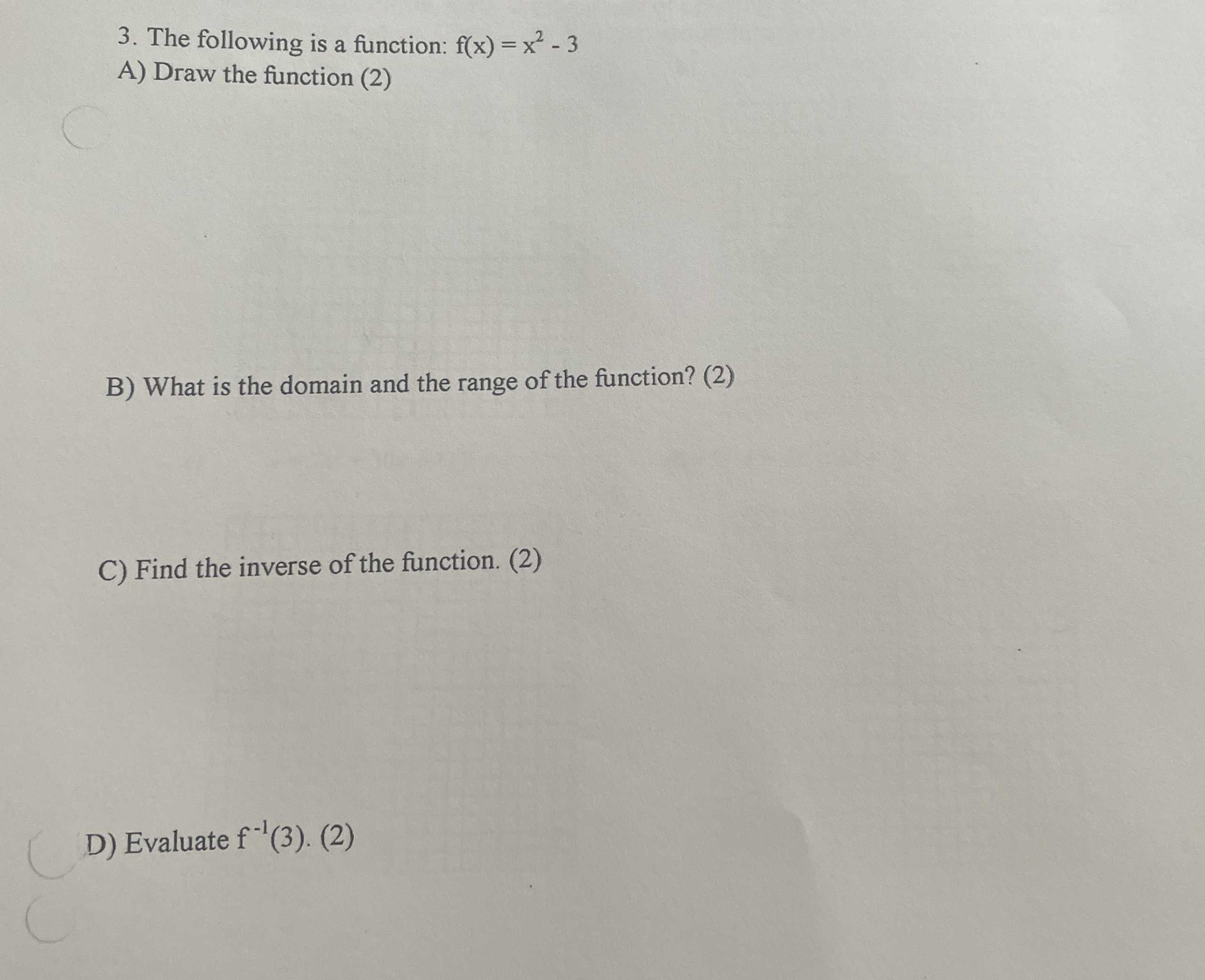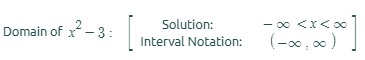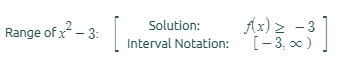### ¿Todavía tienes preguntas de matemáticas?

Pregunte a nuestros tutores expertos
Algebra
Pregunta3. The following is a function: $$f ( x ) = x ^ { 2 } - 3$$

A) Draw the function $$( 2 )$$

B) What is the domain and the range of the function? (2)

C) Find the inverse of the function. (2)

D) Evaluate $$f ^ { - 1 } ( 3 )$$ . (2)$$f^{- 1}(3)= \pm \sqrt{6}$$